Next: Addition of Angular Momentum Up: Spin Precession Previous: Spin Precession

### Exercises

1. Find the Pauli representations of,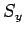, andfor a spin-1 particle.

2. Find the Pauli representations of the normalized eigenstates ofandfor a spin-particle.
3. Suppose that a spin-particle has a spin vector which lies in the-plane, making an anglewith the-axis. Demonstrate that a measurement ofyields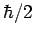with probability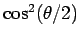, and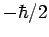with probability.

4. An electron is in the spin-state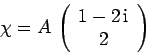in the Pauli representation. Determine the constantby normalizing. If a measurement ofis made, what values will be obtained, and with what probabilities? What is the expectation value of? Repeat the above calculations forand.

5. Consider a spin-system represented by the normalized spinor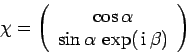in the Pauli representation, where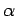andare real. What is the probability that a measurement ofyields?

6. An electron is at rest in an oscillating magnetic field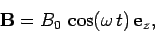whereandare real positive constants.
1. Find the Hamiltonian of the system.
2. If the electron starts in the spin-up state with respect to the-axis, determine the spinor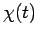which represents the state of the system in the Pauli representation at all subsequent times.
3. Find the probability that a measurement ofyields the resultas a function of time.
4. What is the minimum value ofrequired to force a complete flip in?Next: Addition of Angular Momentum Up: Spin Precession Previous: Spin Precession
Richard Fitzpatrick 2010-07-20